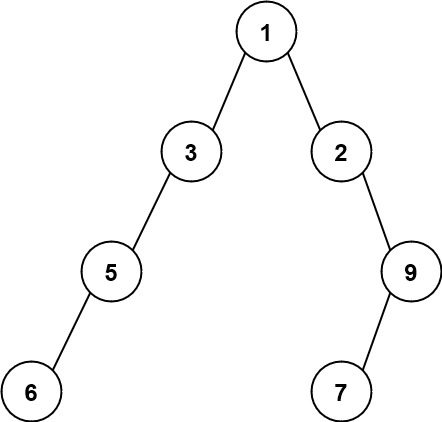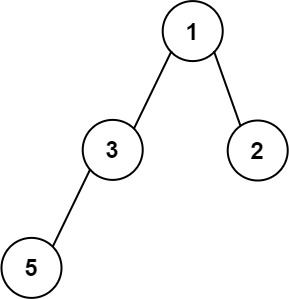# 662. Maximum Width of Binary Tree

Medium
Given the `root` of a binary tree, return the maximum width of the given tree.
The maximum width of a tree is the maximum width among all levels.
The width of one level is defined as the length between the end-nodes (the leftmost and rightmost non-null nodes), where the null nodes between the end-nodes that would be present in a complete binary tree extending down to that level are also counted into the length calculation.
It is guaranteed that the answer will in the range of a 32-bit signed integer.
Example 1:Input: root = [1,3,2,5,3,null,9]
Output: 4
Explanation: The maximum width exists in the third level with length 4 (5,3,null,9).
Example 2:Input: root = [1,3,2,5,null,null,9,6,null,7]
Output: 7
Explanation: The maximum width exists in the fourth level with length 7 (6,null,null,null,null,null,7).
Example 3:Input: root = [1,3,2,5]
Output: 2
Explanation: The maximum width exists in the second level with length 2 (3,2).
Constraints:
• The number of nodes in the tree is in the range `[1, 3000]`.
• `-100 <= Node.val <= 100`

### 解題

/**
* Definition for a binary tree node.
* type TreeNode struct {
* Val int
* Left *TreeNode
* Right *TreeNode
* }
*/
func widthOfBinaryTree(root *TreeNode) int {
m := make(map[int]int) // 存每個 depth 最左邊的 index
ans := 0
var dfs func(*TreeNode, int, int)
dfs = func(root *TreeNode, depth int, index int) {
if root == nil { return }
if _, ok := m[depth]; !ok {
m[depth] = index
}
ans = max(index - m[depth] + 1, ans)
// 如果目前 index 到這個 depth 最左邊 index 的寬度大於答案，更新答案
dfs(root.Left, depth + 1, 2*index)
dfs(root.Right, depth + 1, 2*index + 1)
}
dfs(root, 0, 1)
return ans
}
func max(a, b int) int {
if a > b { return a }
return b
}